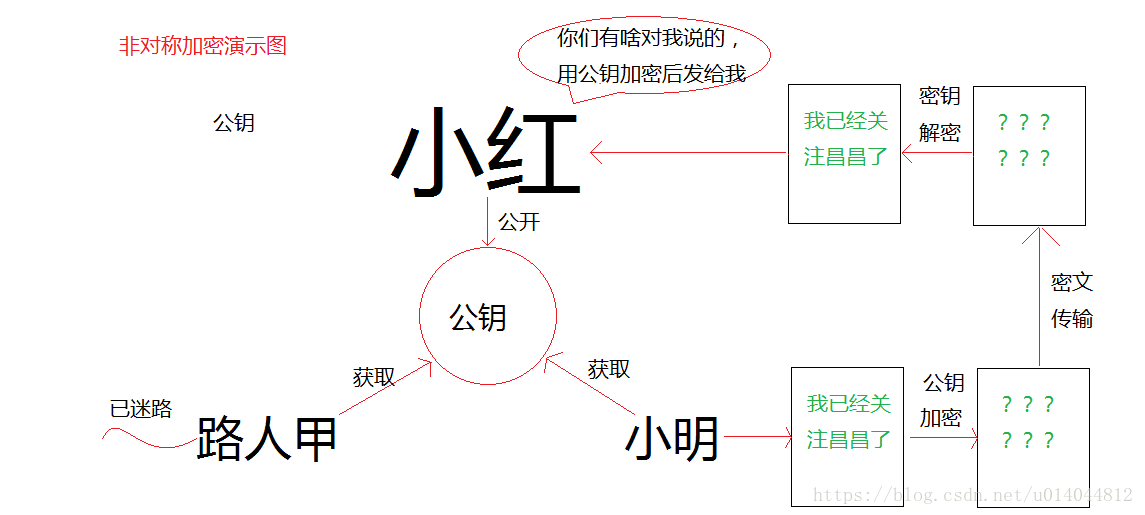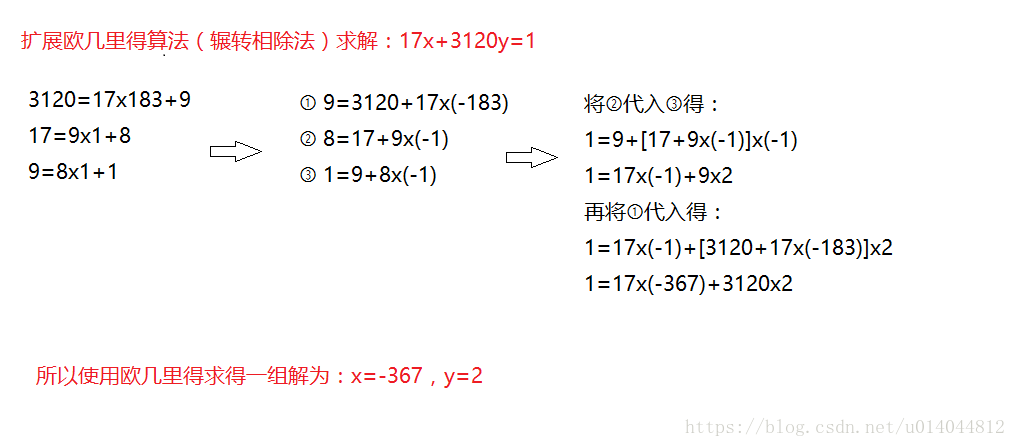ENGLISH 4006-967-446

# RSA加密算法_RSA加解密过程及公式论证(基础数论知识)

### 1、rsa密钥生成过程（1）随机选择两个不相等的质数p和q

（2）计算p和q的乘积n

` n = 61×53 = 3233`

n的长度就是密钥长度，3233写成二进制是110010100001，一共有12位，所以这个密钥就是12位。实际应用中，RSA密钥一般是1024位，重要场合则为2048位。

#### （3）计算n的欧拉函数φ(n)

` 如果n是质数，则 φ(n)=n-1，所以φ(3233) = φ(61x53) = φ(61)φ(53)=60x52=3120`

#### （5）计算e对于φ(n)的模反元素d

` ed≡1(modφ(n))`

`ed–kφ(n)=1`

` 17d–3120k=1`

`17x+3120y=1`` 3和11互质，那么3的模反元素就是4，因为 (3 × 4)-1 可以被11整除。显然，模反元素不止一个， 4加减11的整数倍都是3的模反元素 {…,-18,-7,4,15,26,…}，即如果b是a的模反元素，则 b+kn 都是a的模反元素。`

#### （6）将n和e封装成公钥，n和d封装成私钥

1、p=61; 随机数与q互质

2、q=53;随机数与p互质

3、n=p*q=61*53=3233

4、φ(n)=φ(p*q)=φ(61x53) = φ(61)φ(53)=60x52=3120

5、e=17; 随机数，条件是1< e < φ(n)，且e与φ(n) 互质

6、d=2753; e对于φ(n)的模反元素d

#### （7）rsa算法可靠性

ed≡1 (mod φ(n))。只有知道e和φ(n)，才能算出d

φ(n)=(p-1)(q-1)。只有知道p和q，才能算出φ(n)

n=pq。只有将n因数分解，才能算出p和q

123018668453011775513049495838496272077285356959533479219732245215172640050726

365751874520219978646938995647494277406384592519255732630345373154826850791702

6122142913461670429214311602221240479274737794080665351419597459856902143413

33478071698956898786044169

84821269081770479498371376

85689124313889828837938780

02287614711652531743087737

814467999489

×

36746043666799590428244633

79962795263227915816434308

76426760322838157396665112

79233373417143396810270092

798736308917

1999年，RSA-155 (512 bits)被成功分解，花了五个月时间（约8000 MIPS年）和224 CPU hours在一台有3.2G中央内存的Cray C916计算机上完成。

2009年12月12日，编号为RSA-768（768 bits, 232 digits）数也被成功分解。这一事件威胁了现通行的1024-bit密钥的安全性，普遍认为用户应尽快升级到2048-bit或以上。

### 2、rsa加解密演示

（1）加密要用公钥 (n,e)

` me≡c(modn)`

`6517≡c(mod3233)`

（2）解密要用私钥(n,d)

`cd≡m(modn)`

`27902753≡m(mod3233)`

### 3、rsa公式论证

p： 随机数与q互质

q：随机数与p互质

n=p*q

φ(n)=φ(p*q)=φ(p)*φ(q)=(p-1)(q-1)

e： 随机数，条件是1< e < φ(n)，且e与φ(n) 互质

d：e对于φ(n)的模反元素d：ed≡1 (mod φ(n))

m：小明发送的明文

c：小明用公钥加密后的密文

` 解密公式：cd≡m(modn)`

`  加密公式：me≡c(modn)→c=me–kn`

` (me–kn)d≡m(modn)`

`med≡m(modn)`

`(2–2n)2=4−8n+4n2`

`ed≡1(modφ(n))→ed=hφ(n)+1`

`mhφ(n)+1≡m(modn)`

（1） m与n互质

aφ(n)≡1(modn)

`mφ(n)≡1(modn)→mφ(n)=1+kn→(mφ(n))h=(1+kn)h`

`(mφ(n))h=(1+kn)h≡1(modn)`

`(mφ(n))h≡1(modn)→(mφ(n))h=1+kn→(mφ(n))h∗m=(1+kn)∗m`

`(mφ(n))h∗m=(1+kn)∗m≡m(modn)→mhφ(n)+1≡m(modn)`

（2） m与n不是互质关系

(kp)φ(q)≡1(modq)→(kp)q−1≡1(modq)

`[(kp)q−1]h(p−1)×kp≡kp(modq)→(kp)h(p−1)(q−1)+1≡kp(modq)`

`ed≡1(modφ(n))→ed=hφ(n)+1→ed=h(p−1)(q−1)+1`

`(kp)ed≡kp(modq)→(kp)ed=tq+kp`

`(kp)ed=rpq+kp`

` med=rn+m→med≡m(modn)`

`ed≡1(modφ(n))→ed=hφ(n)+1`

`mhφ(n)+1≡m(modn)`

### 相关资讯

SHA-1和SHA-2算法有什么区别？

SHA是一个密码散列函数家族，是FIPS所认证的安全散列算法。能计算出一个数字消息所对应到的，长度固定的字符串（又称消息摘要）的算法。且若输入的消息不同，它们对应到不同字符串的机率很高。SHA-1和SHA-2是该算法不同的两个版本，它们的构造和签名的长度都有所不一样，但可以把SHA-2理解为SHA-1的继承者。

### 最新资讯

Hash算法的特点、应用和实现方法详解

rsa算法产生的过程与原理详解

iphone手机如何安装ssl证书?